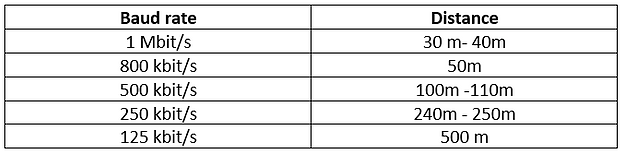# What is the Baud rate?

The rate at which the information is transferred in a communication channel is known as the baud rate. Or, more generally, the baud rate is the speed/rate at which the data is transmitted on the network. This is expressed in bits per second. Hence the number of bits that travel on a communication network in a second is the baud rate.

For a CAN bus, which is a serial, multi-master bus, if the baud rate is mentioned as “500000 baud”, that port on CAN can transfer a maximum of 500000 bits per second.

Different Baud-rates supported on CANbus:

• 125 kbps

• 250 kbps

• 500 kbps (Standard for most automotive applications)

• 1000 kbps (1mbps)

Various software allows the user to set the bus speed depending upon the need of the CANbus. The below image is from Influx’s DiaLog software, enabling the user to select the bus speed (from various available options).## How many messages does every baud rate support?

CANbus supports the transmission of data that can be 8 Byte long. But, the transmission of such long messages results in larger latency.  At a bit rate of about 1 Mbit/s, with an average data length of 4 bytes, up to 10,000 messages per second can be transmitted using a standard format, i.e., with 8 bytes data length, up to 7,200 messages can be sent per second.

Using simple formulas, one can calculate the approx—time to transfer one frame on the CAN bus.

Now, for example, 1 CAN frame contains approximately 125 bits.

1. Assuming bit rate = 250kBits/psec.

bit time = 1 / bit rate = 1 / (250 * 1000) s = 4 * s = 4 µs.

Therefore, 1 bit will take 4 µs to transfer on the bus when using 250 kBit/s.

So the approximate time to transfer 1 frame is (4 µs/bit * 125 bit) = 500 µs.

2. Assuming bit rate= 500 kBit/s.

bit time = 1 / bit rate = 1 / (500 * 1000) s = 2 * s = 2 µs

Therefore, 1 bit will take 2µs to transfer on a bus when using 500 kBit/s.

So, the approximate time to transfer 1 frame is (2 µs/bit * 125 bit) = 250 µs.

## The formula for calculating Bus load for CAN bus:

Bus load is calculated as a percentage.

Bus load = #bytes send / speed

Bus load % = bytes (to be send in a second) * (8bits) / (baud rate bps) * 100%

Assuming the bytes to be sent in a second as 1000

So, at 250kbps, the bus load % will become: -

1000*8/250000*100% = 3.2%

Similarly, at 500kbps, the bus load % will become: -

1000*8/250000*100% = 1.6%

However, at higher message priority, bus use of 70 to 80% can typically be achieved.

To transmit a given number of frames on the bus in a given number of seconds. The average bits per message (32-bit data) is assumed to be 76 unless defined (it may vary).

• 5ms -5 frames

1/ 0.005 * 5 * 76 = 760,00

• 10ms -5 frames.

1 / 0.010 * 5 * 76 = 38,000

• 100ms -5 frames

1 / 0.100 * 5 * 76 = 3800

Total number of frames = 760,00 + 38,000 + 3,800 = 117,800

Bus Load at baud rate 250 kbps = 117,800/250000*100% = 47.12%

Bus Load at baud rate 500 kbps = 117,800/500000*100% = 23.56%

In the below, screenshot one can see the active logging, trace viewer for Influx’s Rebel CT/LT, Channel (HS). Parameters such as Bitrate and Busload (total, per second and load) can be viewed here. Also, Rx/Tx messages and the error frames (total, per second and load) can be easily viewed.How it affects the length of the CAN bus?

For a CAN bus, the baud rate and the length of the cable are inversely proportional—the higher the baud rate lesser the length of the cable.